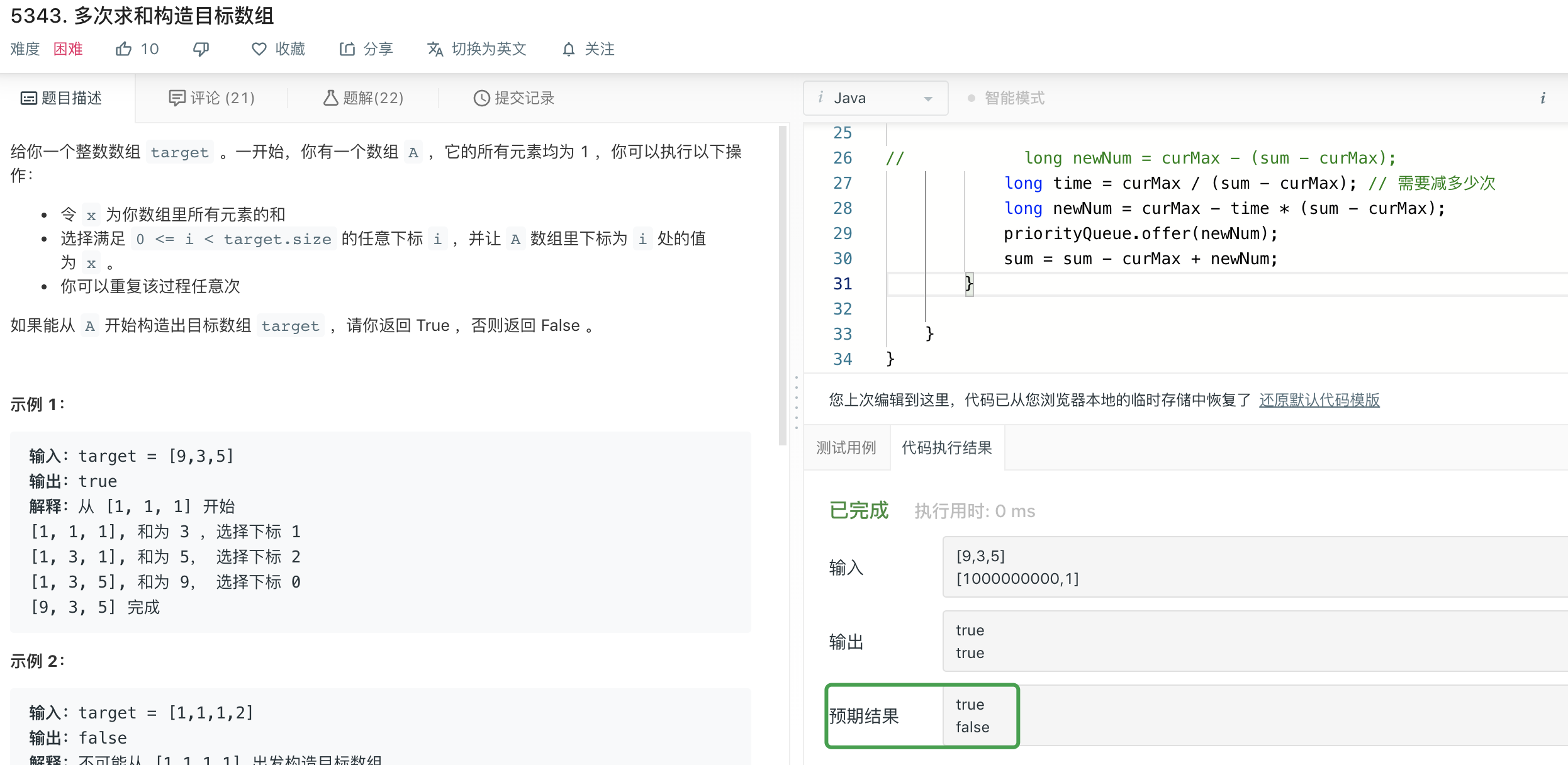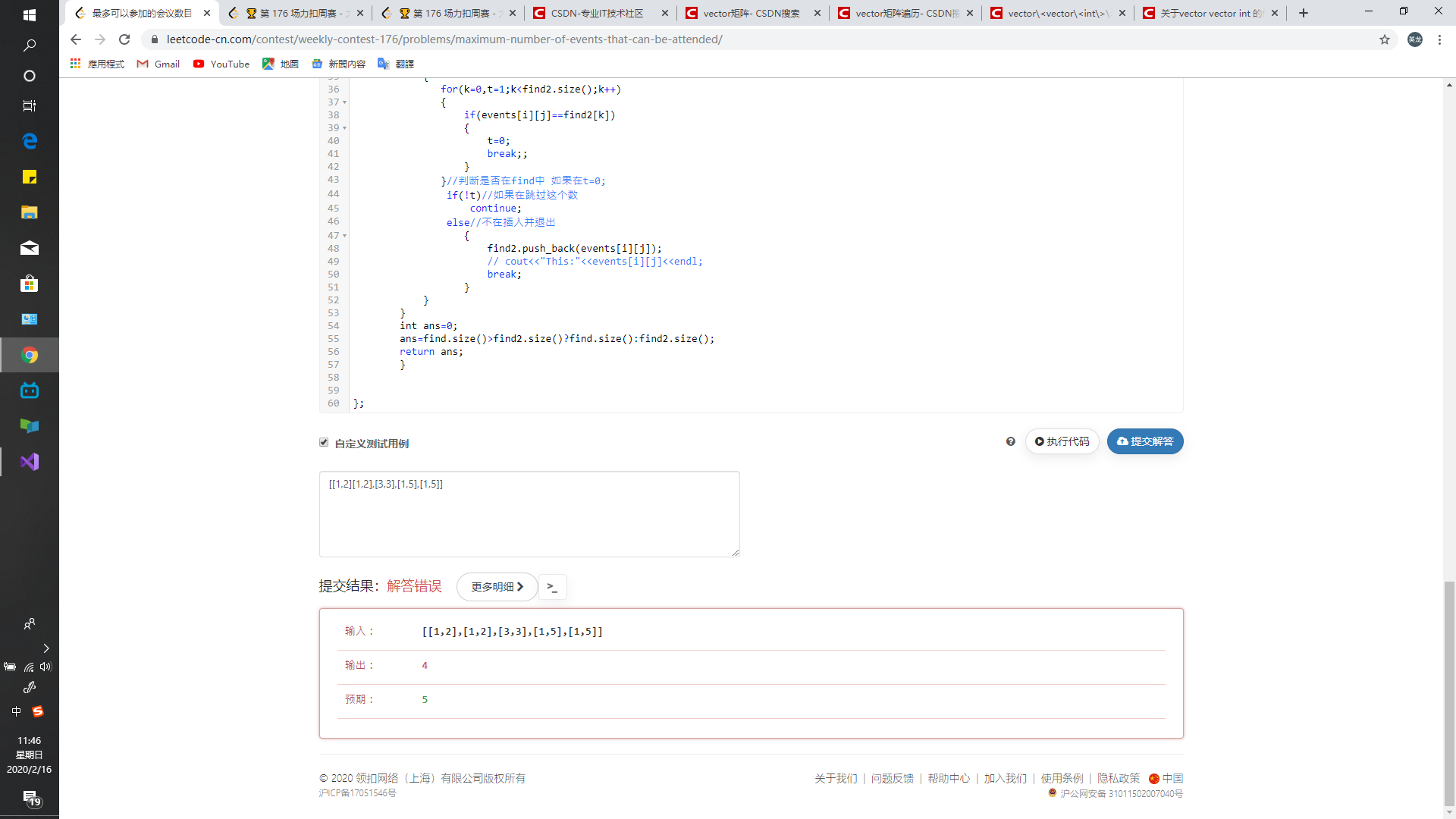🏆 第 176 场力扣周赛

class Solution {
public:
int countNegatives(vector<vector<int>>& grid) {
int n = grid.size();
int m = grid.size();
int ret = 0;
for (int i = 0;i < n;i++) {
for (int j = 0;j < m;j++) {
if (grid[i][j] < 0) {
ret++;
}
}
}
return ret;
}
};


• 解法一：

class ProductOfNumbers {
public:

vector<vector<int>> cache;

ProductOfNumbers() {
cache.clear();
cache.push_back(vector<int>(101, 0));
}

vector<int> t = cache[cache.size() - 1];
t[num]++;
cache.push_back(t);
}

int getProduct(int k) {
vector<int> t = cache[cache.size() - 1];
for (int i = 0;i <= 100;i++) {
t[i] -= cache[cache.size() - 1 - k][i];
}
if (t > 0) {
return 0;
}
int ret = 1;
for (int i = 2;i <= 100;i++) {
for (int j = 0;j < t[i];j++) {
ret *= i;
}
}
return ret;
}
};

• 解法二：

class ProductOfNumbers {
public:

vector<vector<int>> cache;
int lastZero;
int index;

ProductOfNumbers() {
cache.clear();
lastZero = -1;
index = 0;
}

if (num == 0) {
lastZero = index;
} else if (num > 1) {
cache.push_back({index, num});
}
index++;
}

int getProduct(int k) {
int n = index;
if (lastZero >= n - k) {
return 0;
}
int ret = 1;
for (int i = (int)cache.size() - 1;i >= 0 && cache[i] >= n - k;i--) {
ret *= cache[i];
}
return ret;
}
};

• 解法三：

class ProductOfNumbers {
public:

vector<int> cache;

ProductOfNumbers() {
cache.clear();
cache.push_back(1);
}

if (num == 0) {
cache.clear();
cache.push_back(1);
} else {
cache.push_back(cache[cache.size() - 1] * num);
}
}

int getProduct(int k) {
int n = cache.size() - 1;
if (n - k < 0) {
return 0;
} else {
return cache[n] / cache[n - k];
}
}
};


class Solution {
public:
int maxEvents(vector<vector<int>>& events) {
priority_queue<int, vector<int>, greater<int>> q;

sort(events.begin(), events.end());
int ret = 0;
int last = 1;
int i = 0;
while (i < events.size() || q.size() > 0) {
while (i < events.size() && events[i] == last) {
q.push(events[i]);
i++;
}
while (q.size() > 0 && q.top() < last) {
q.pop();
}
if (q.size() != 0) {
q.pop();
ret++;
}
last++;
}
return ret;
}
};


n 轮后，最大值 p 替换成 $p - n * (s - p)$，数组的和替换成 $p - (n - 1) * (s - p)$

class Solution {
public:
bool isPossible(vector<int>& target) {
if (target.size() == 1) {
if (target == 1) {
return true;
} else {
return false;
}
}
priority_queue<int> q;
long long s = 0;
for (int i = 0;i < target.size();i++) {
q.push(target[i]);
s += target[i];
}
while (1) {
if (q.top() == 1) {
return true;
}
long long p = q.top();
q.pop();
if (q.top() == 1) {
if ((p - 1) % (s - p) == 0) {
return true;
} else {
return false;
}
} else {
long long n = (p - q.top()) / (s - p) + 1;
long long x = p - (s - p) * n;
s = p - (s - p) * (n - 1);
if (x <= 0) {
return false;
}
q.push(x);
}
}
return false;
}
};


19

T1: 签到题

T2:

T3:

T4

1. 先找出最大的数。 若最大的数为1， 返回true.
2. 将最大的数减去其他所有数之和。如果该数为0或者负， 返回false.
3. 将原来最大的数用2中得到数替换。

@ottff 提出如果数据是[1e9, 1]的话， 会超时。 因此， 记录最大和次大数。 每一次看最大的数能够减多少次会比次大数小。 然后一并减掉就可以了。 这种一定要注意边界情况。

13

9

[1000000000,1]

8

T2 不同语言的超时标准有问题吧，有的语言暴力都可以过

6

#### 5342. 最多可以参加的会议数目

public int maxEvents(int[][] events) {
// 会议按照开始时间排序
Arrays.sort(events, (e1, e2) -> Integer.compare(e1, e2));

// 优先队列按照结束时间排序
PriorityQueue<int[]> pq = new PriorityQueue<>((e1, e2) -> Integer.compare(e1, e2));

int N = events.length;
int i = 0;
int d = 1;
int count = 0;
while (i < N || !pq.isEmpty()) {
// 模拟第 d 天的决策
// 将已经开始的会议加入队列
while (i < N && events[i] <= d) {
i++;
}

// 找出一个可以参加的最早结束的会议
while (!pq.isEmpty()) {
int[] e = pq.poll();
if (e >= d) {
count++;
break;
}
}
d++;
}

return count;
}

4

3
221